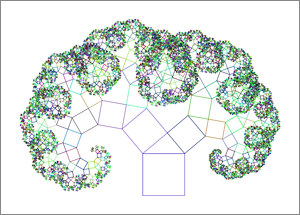# Small TikZ Drawing of a Pythagoras Tree

Posted on 2013-06-27 23:23 by Timo Bingmann at Permlink with 0 Comments. Tags: fun

This post just contains a simple TikZ/LaTeX program to construct a Pythagoras tree. I wrote it to figure out how to write recursive TikZ drawings. The rest is rather pretty.

% Draw Pythagoras Trees using TikZ
% Author: Timo Bingmann <tb@panthema.net>, 2013-06-27
\documentclass[a4paper]{article}

\usepackage{fullpage}
\usepackage{tikz}
\usepackage{animate}

% recursively draw a Pythagoras Tree fractal
% \PythagorasTree{levels}{angle}
\newcommand{\PythagorasTree}{%
\ifnum#1=0\else
% randomly pick a color, prefer green and blue shades
\pgfmathsetmacro{\r}{0.6*rnd}
\pgfmathsetmacro{\g}{0.9*rnd}
\pgfmathsetmacro{\b}{0.7*rnd}
\definecolor{MyColor}{rgb}{\r,\g,\b}

% draw the rectangle of this level
\draw[draw=MyColor] (0,0) rectangle (1,1);

% decrement level counter
\pgfmathtruncatemacro{\next}{#1-1}

% transform scope for left branch: move origin (1cm,0cm), rotate and scale
% with the length of the left cathetus.
\begin{scope}[
yshift=1cm,xshift=0cm,
rotate=#2,scale={cos(#2)}
]
\PythagorasTree{\next}{#2}
\end{scope}

% now for the tricks: transform scope for right branch: move origin to the
% top point of the triangle, rotate reverse and scale with length of right
% cathetus.
\begin{scope}[
yshift={1cm * (1 + sin(#2)*cos(#2))},
xshift={1cm * (cos(#2)*cos(#2))},
rotate={#2-90},scale={sin(#2)}
]
\PythagorasTree{\next}{#2}
\end{scope}
\fi
}

\begin{document}

\parindent=0pt

% draw just a single Pythagoras Tree
\begin{tikzpicture}[scale=1.5]
% recursively draw tree
\PythagorasTree{13}{40}
\end{tikzpicture}
\bigskip\bigskip

% macro to draw and annotate a Pythagoras Tree
\newcommand{\MakeTree}{%
% minipage to keep things together
\begin{minipage}{\linewidth}
\textbf{Pythagoras Tree, order #1, angle #2:}
\bigskip

\begin{center}
\begin{tikzpicture}[scale=1.5]
% recursively draw tree
\PythagorasTree{#1}{#2}
#3 % add some extra tikz code
\end{tikzpicture}
\end{center}
\bigskip

\end{minipage}
}

% draw some more trees with different parameters
\iftrue
\MakeTree{13}{38}{}
\MakeTree{11}{45}{}
\MakeTree{11}{30}{}
\MakeTree{11}{65}{}
\fi

% draw animated growing Pythagoras Tree
\iftrue
\clearpage
The following frame is an animated growing Pythagoras Tree, properly
\end{document}